# Water density

Jump to: navigation, search

## Formation water density

The density of formation water (brine) at standard conditions (14.7psia & 60°F):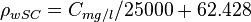$\rho_{wSC} = C_{mg/l}/25000 + 62.428$

The density of formation water (brine) at reservoir conditions: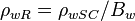$\rho_{wR}=\rho_{wSC}/B_w$

## Example. Calculating formation water density

Example source 

### Input data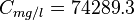$C_{mg/l} = 74289.3$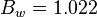$B_w = 1.022$ at 3176 psia and 165°F

Estimate the density of the brine at standard conditions (14.7psia & 60°F) and at 3176 psia and 165°F?

### Solution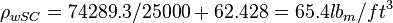$\rho_{wSC} = 74289.3/25000 + 62.428 = 65.4 lb_m/ft^3$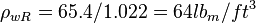$\rho_{wR} = 65.4/1.022= 64 lb_m/ft^3$

The solution is also available in the PVT software model at [The solution is available in the PVT calculator software model at www.pengtools.com

## Nomenclature$B_w$ = water formation volume factor, res bbl/STB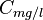$C_{mg/l}$ = water salinity or solids concentration, mg/l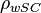$\rho_{wSC}$ = water density at standard conditions, lbm/ft3

## See also

Water bubble point pressure
Water compressibility
Water density
Water formation volume factor
Water salinity from density equation
Water solids concentration
Water viscosity
Gas/Water Interfacial Tension